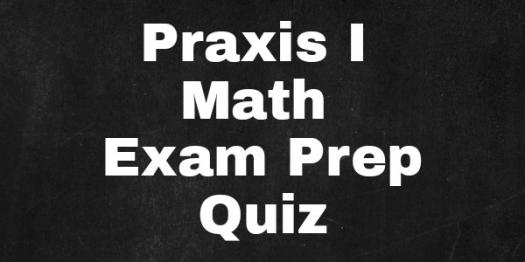# PRAXIS 1 Math Exam Prep

10 Questions | Total Attempts: 107SettingsThe Praxis 1 Maths Exam is an highly regarded one. One which you need painstakingly prepare for. Maths skills are tested as well as the competence to teach students. There is a need to be merge accuracy with speed also as well as efficiency which forms the reason for the limit in time as well as the required pass mark. Don't be caught in the web by taking this quiz.

• 1.
Which of these disciplines does not employ statistical principles?
• A.

Astronomy

• B.

Computational biology

• C.

Data mining

• D.

Actuarial science

• 2.
When did the study of statistics start?
• A.

1654

• B.

1667

• C.

1678

• D.

1663

• 3.
When was the method of least squares first described?
• A.

1805

• B.

1809

• C.

1867

• D.

1871

• 4.
Which of these mathematicians is associated with probability theory?
• A.

Pierre de Fermat

• B.

Blaise Pascal

• C.

Isaac Newton

• D.

Gerolamo Cardano

• 5.
What is most common observation in a data set called?
• A.

Median

• B.

Range

• C.

Mean

• D.

Mode

• 6.
Which of these is not an assumption in algebra?
• A.

Associative assumption

• B.

Unital assumption

• C.

Unitary assumption

• D.

Commutative assumption

• 7.
What branch of algebra use truth values?
• A.

Computer algebra

• B.

Commutative algebra

• C.

Computer algebra

• D.

Boolean algebra

• 8.
Which of these operations comes first in a mathematical problem?
• A.

Division

• B.

Multiplication

• C.

• D.

Subtraction

• 9.
Which of these is not a modern presentation of geometry?
• A.

Tangents

• B.

Planes

• C.

Rotations

• D.

Lines

• 10.
When was determinants first used for solving matrices problems?
• A.

1768

• B.

1637

• C.

1789

• D.

1789

Related TopicsBack to top